# Geometry Worksheets

#### What is Geometry?

It is an integral branch of mathematics that defines the objects' sizes, angles, shapes, measurements, and dimensions. This domain includes the study of squares, triangles, and circles as they come under the category of flat shapes. We can call them 2D (two-dimension) shapes. There are also three-dimensional (3D) objects as it includes the height and depth of things. Usually, we study these shapes for solid objects, or we can say that the study of 3D shapes in mathematics is the study of solid shapes in geometry. These categories have further parts, such as the shapes' angle, congruence, and the coordinate plane. Angle – It is the point where two edges of the shape joined as the object's vertex that makes an angle. Different angles of various shapes in geometry have different measures. In geometry, there is a different measurement of shapes as per their categories. The shapes like square, quadrilateral, rectangle, and four-sided shapes have an interior angle’s degree that is 360. Polygons are also part of the geometric domain. It has different shapes according to the sides of the shapes like a pentagon (a shape with five sides), hexagon (a shape with six sides), heptagon (a shape with seven sides), and an octagon (shapes with eight sides), etc. The size and measurement of varies with the estimated figure of the angle.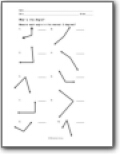###### Angles

Students will learn how to classify, name, and measure angles.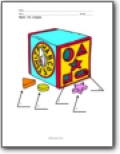###### Geometric Shapes

Students will learn the names of shapes, how to recognize congruent shapes, and understand shape transformations.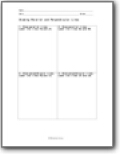###### Points and Lines

Students will begin to understand how to draw lines, rays, and classify pairs of lines.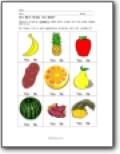###### Symmetry

We introduce the concept and apply it to shapes and everyday objects.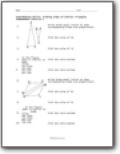We learn how to draw and classify them. We also start to find unknown sides. The concept similarity is also reviewed.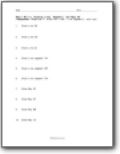###### Drawing Lines, Segments, and Rays

Students will draw a described line, ray, or segment as instructed.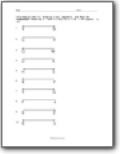###### Identification

Identify each as a line, line segment, or ray.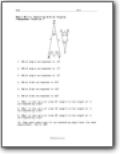###### Exploring Similar Figures

Students will find corresponding angles and sides by using a fixed triangle.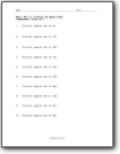###### Finding the Square Root

Students will determine the square root of given numbers.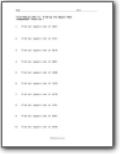###### Find the Square Root of Large Numbers

Given a value of a sizable number, students will determine the square root.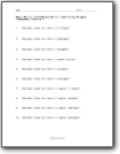###### Identifying Polygons Based on Sides

Given a number of sides, students will name polygons.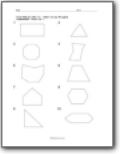###### Identifying Drawn Polygons

You will see a shape that is drawn and you need to name it.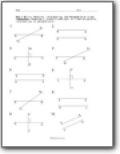###### Parallel, Intersecting, and Perpendicular Lines

Classify each pair of lines as parallel, intersecting, or perpendicular.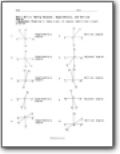###### Naming Adjacent, Supplementary, and Vertical Angles

Name a pair of angles identified in each problem.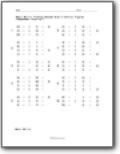###### Finding Unknown Side in Similar Figures

Given that you are working with similar figures, find the missing sides.###### Finding Unknown Side in Similar Figures

We work with larger side ratios in this pack.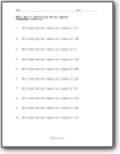###### Determining Perfect Squares

Students will rewrite each value as a perfect square.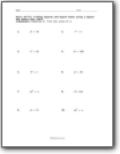###### Finding Squares and Square Roots using a Square and Square Root Table

Find the missing value in each situation.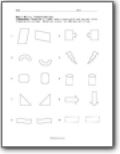###### Transformations

Label each figure with the correct term: Translation (slide), Rotation (turn), or Reflection (flip).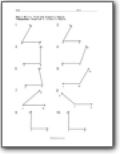###### Find and Classify Angles

Given a drawn angle, classify it based on your visual estimate of the angle.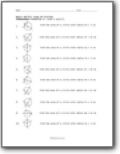###### Area of Circles

Find the area of a circle with a given radius.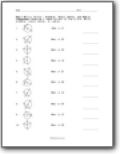###### Diameter, Chord, Center, and Radius

Name the part of the circle. Write diameter, chord, center, or radius.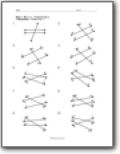###### Transversals

Name the transversal line in each instance.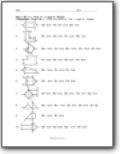###### Area of Irregular Shapes

Find the area of the irregular shapes.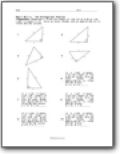###### The Pythagorean Theorem

Find the length of the third side of the right triangle. Give an exact answer and an approximation to three decimal places.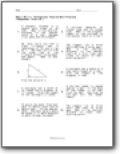###### Pythagorean Theorem Word Problems

Solve a series of word based problems that apply the Pythagorean Theorem. Example problem: A baseball diamond is a square with sides of 80 feet. What is the shortest distance to the nearest tenth of a foot, between first base and third base?

### How is Geometry Used in the Real World?

Math might look like a difficult subject, but in reality, it is quite interesting, and there are several things that are quite easy to understand in the subject. One of the best examples that can be taken to explain why it is so fun is geometry. Do you know what it is? Let us take a look! Geometry is a branch of math that includes shapes and sizes, their measurements, their areas, their volumes and everything else that constitutes them. Studying geometry is really fun because it involves a series of shapes that come in all sizes. From a simple circle to a complicated shape of the building, everything we see includes geometry. Now, if you are wondering how geometry is used in the real world, the answer is quite simple. It is used in the architecture of big buildings, used to measure an object, computer-aided design for construction of blueprints, the design of assembly systems in manufacturing, nanotechnology, computer graphics, visual graphs, video game programming and virtual reality creation. Like we said, everything involves geometry!